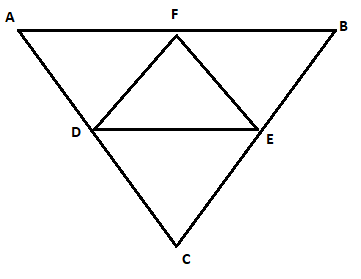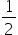Mathematics
Easy

Question

# In a triangle ABC - D, E, F are mid points.If AB = 3x +15, DE = 5x + 4, what is the length of AB?

## 24161821Hint:

## The correct answer is: 18

### Step 1 of 1:We have triangle ABC and DEFNow, using midpoint theorem we can thatDE =ABAB = 2 DE3x + 15 = 2(5x + 4)3x + 15 = 10x + 87x = 7x=1AB = 3(1) + 15 = 18

Linear equation in one variable can be solved using fundamental operations

### Related Questions to study#### With Turito Foundation.#### Get an Expert Advice From Turito.